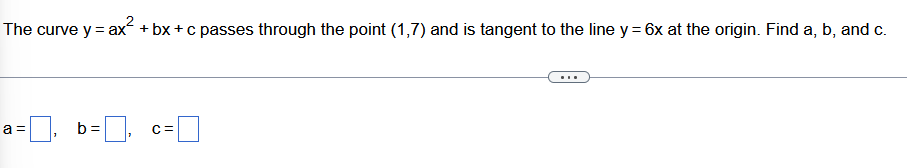# Question Solved1 AnswerThe curve y = ax +bx+c passes through the point (1,7) and is tangent to the line y = 6x at the origin. Find a, b, and c. a = b= C= The curve y = ax +bx+c passes through the point (1,7) and is tangent to the line y = 6x at the origin. Find a, b, and c. a = b= C=Transcribed Image Text: The curve y = ax +bx+c passes through the point (1,7) and is tangent to the line y = 6x at the origin. Find a, b, and c. a = b= C=
More
Transcribed Image Text: The curve y = ax +bx+c passes through the point (1,7) and is tangent to the line y = 6x at the origin. Find a, b, and c. a = b= C=